# 588x and 488x Execution Time Speedups of a Volcanic Hazard Assessment Code

Thursday, November 5, 2020

The Probabilistic Volcanic Hazard Assessment Work Flow package (PVHA_WF) is a workflow created for the ChEESE CoE Pilot Demonstrator 6 (PD6). It is used to simulate short-term volcanic hazards and the risk of hazardous volcanic particles presence at different flight levels over geographical areas. Figure 1 shows such areas with different levels of tephra concentration.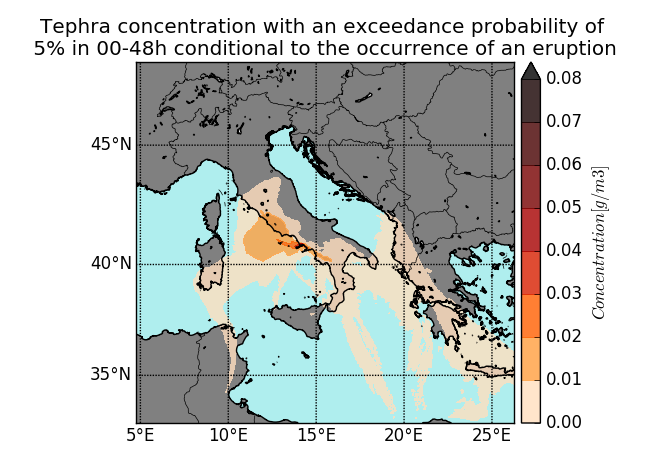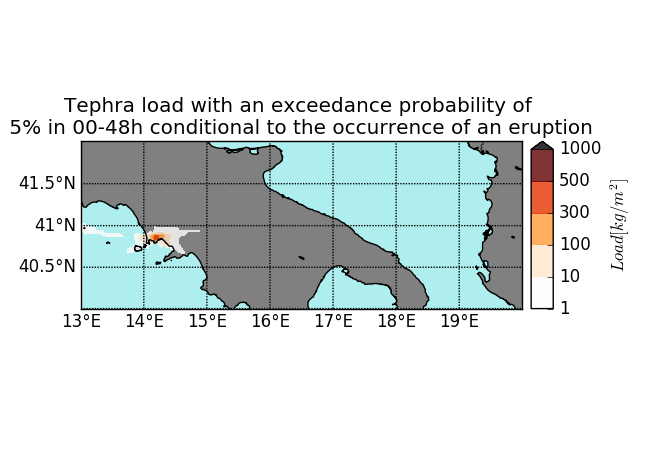Figure 1: Fly 0 and ground level simulations of PVHA_WF

Due to recent code modifications, one task of the workflow became critical, taking up an unacceptable amount of time to complete. A decision was made to separate this task into an independent application, resulting into a Python + MPI script in charge of computing statistical probabilities from a Fall3D output which is shown in Figure 2. The resulting application proved to be extremely slow and unable to run even on the smallest test cases. Subsequently, in collaboration with the code deveolpers from the ChEESE CoE, we conducted a POP performance assessment of this application with the goal to prepare it for production runs.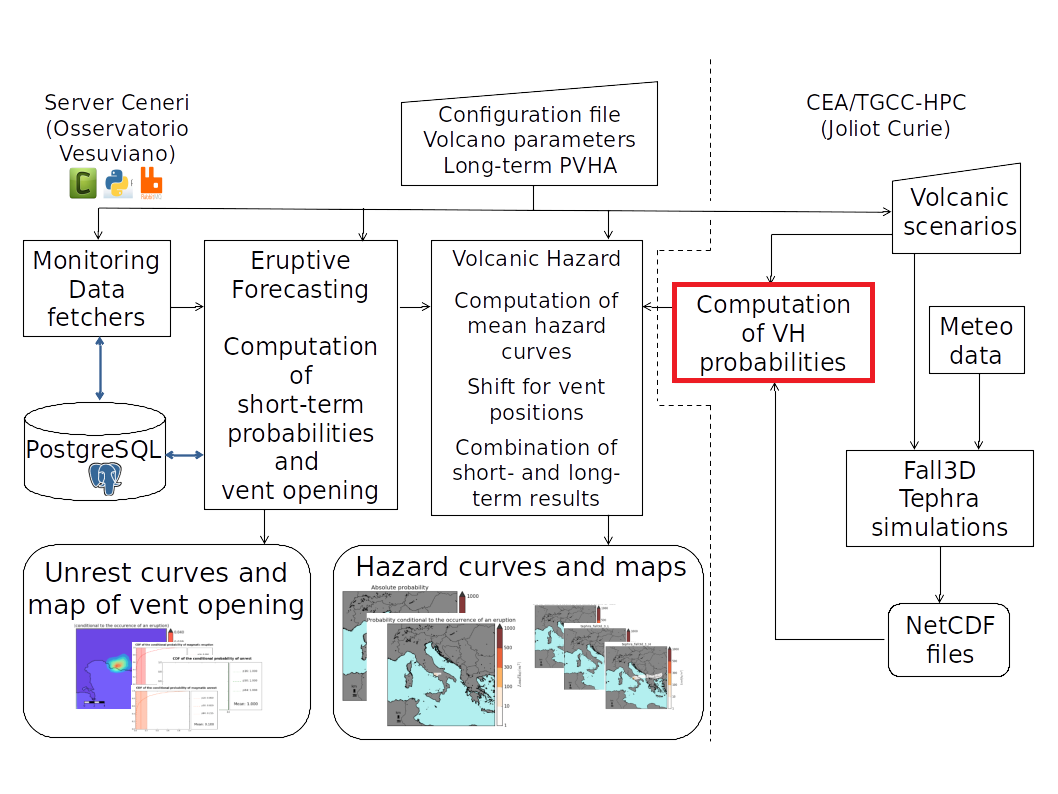Figure 2: PVHA_WF workflow diagram. The critical task is highlighted with a red square

Following the POP methodology, we started this service with a performance analysis. Firstly, we discussed with the developer the application’s performance issues, from where we concluded that we needed to analyse 2 execution configurations (ground and fly 0 levels). Then, we provided the developers with the knowledge of how to trace the Python + MPI application on the target cluster using our tracing tools. We analysed the resulting traces, produced the POP metrics, studied the root cause of each problem highlighted by our metrics, and generated report slides.

The POP metrics for the ground level use case are shown in Table 1.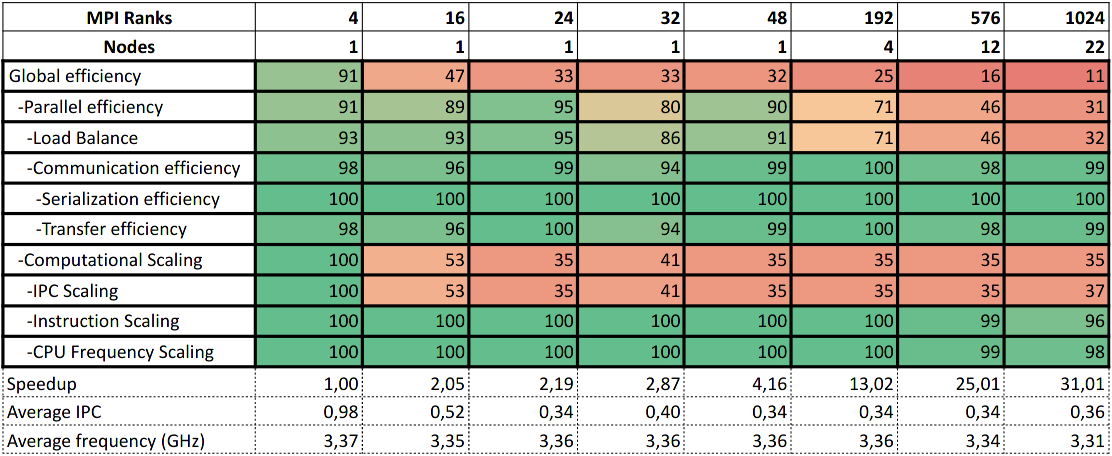Table 1: POP metrics for PVHA_WF ground level use case

From the numbers, we can conclude that load balance and IPC degradation prevent the application from scaling with processes. We found that the load balance problems are caused by a sequential region not parallelized with MPI. The IPC problems were caused by bad code and memory congestions.

The POP metrics for the fly 0 level use case are shown in Table 2.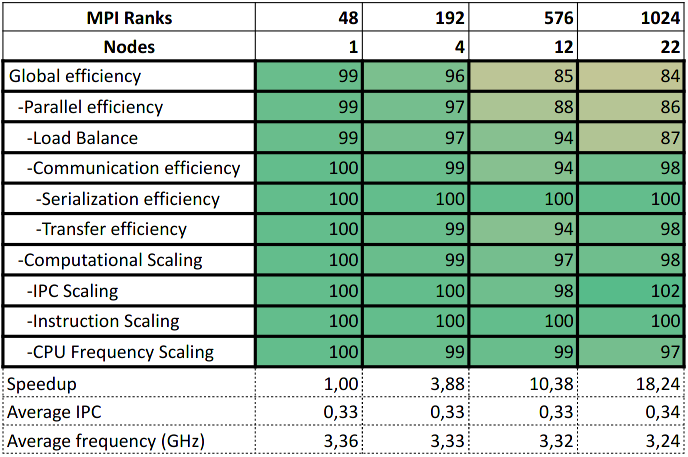Table 2: POP metrics for PVHA_WF fly 0 level use case

For this use case, parallel efficiency is excellent making the code’s scalability acceptable. However, the very low average IPC leaves room for improvement. Both configurations show slow sequential code and Python’s runtime takes a large number of instructions for solving the problem.

Once we identified the root causes of the performance problems, we agreed with the user to address them using our POP Proof of Concept (PoC) service. We fixed the poor IPC and inefficient sequential code by refactoring the 2 main compute functions (Step1 & Step2, taking 98% of total runtime) using Numpy vectorization techniques. Already just with this optimization we noticed how those functions were 775 times faster on one CPU using a test input case. Despite reducing sequential execution time by a factor of hundreds, parallel efficiency was still a problem. With high number of processes the non-parallelized function (Step2) dominated the runtime. Therefore, we parallelized it using the mpi4py Python package.

After our PoC, the PVHA_WF application was 588 and 488 times faster with fly 0 and ground levels, respectively, as shown in Figure 3.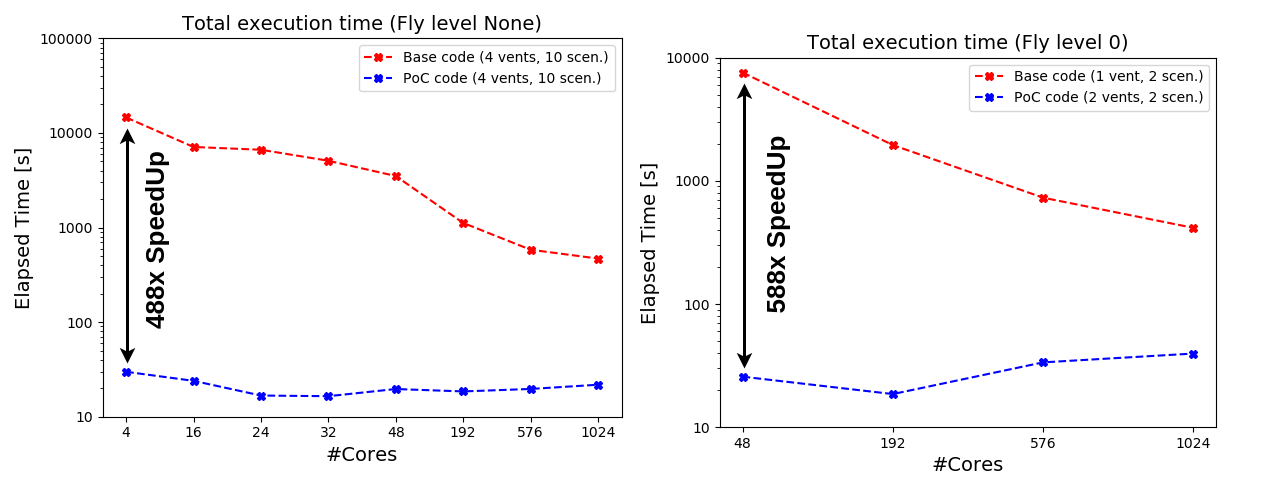Figure 3: PVHA_WF total execution time comparison

To get gain further insight, we measured the gain of each optimized function separately as well as their new average IPC. We observed that a huge speedup of 2039x was obtained with Step1. The average IPC of Step1 almost doubled, while of Step2 decreased slightly but is still maintaining good values as shown in Figure 4.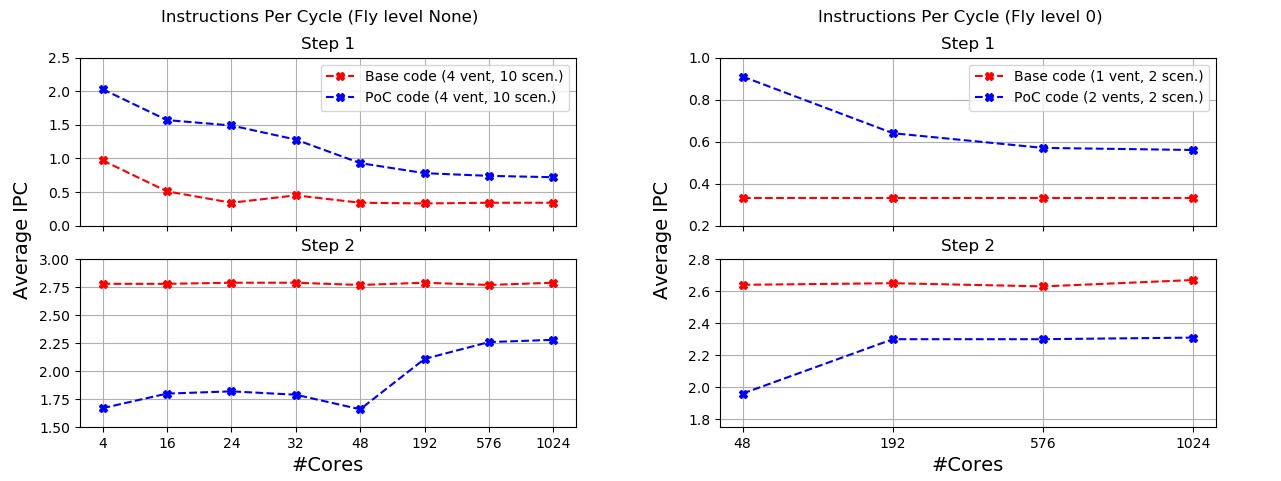Figure 4: PVHA_WF average IPC comparison of each optimized functionFigure 5: PVHA_WF execution time comparison of each optimized function

In conclusion, we took the PVHA_WF Python + MPI application and achieved speedups of 588 and 488 times faster as shown in Figure 3. First, our performance assessment service identified the major performance problems: non-parallelized regions and extremely poor sequential performance. Second, our proof-of-concept service addressed those problems: we parallelized the sequential code with MPI and refactored the application with Numpy vectorization. In the end, our POP methodology succeeded by producing an application suitable for HPC.

-- Oleksandr Rudyy (HLRS)